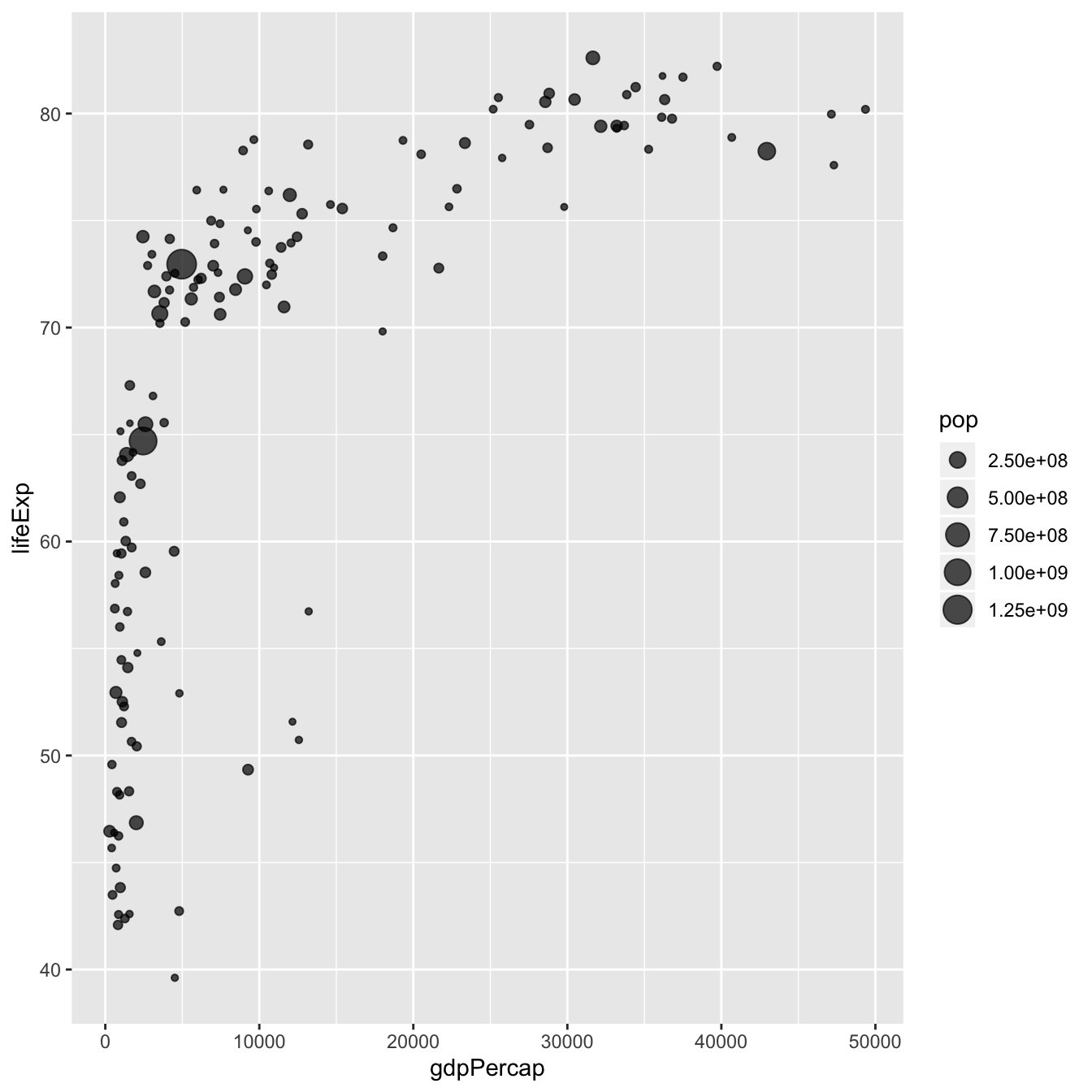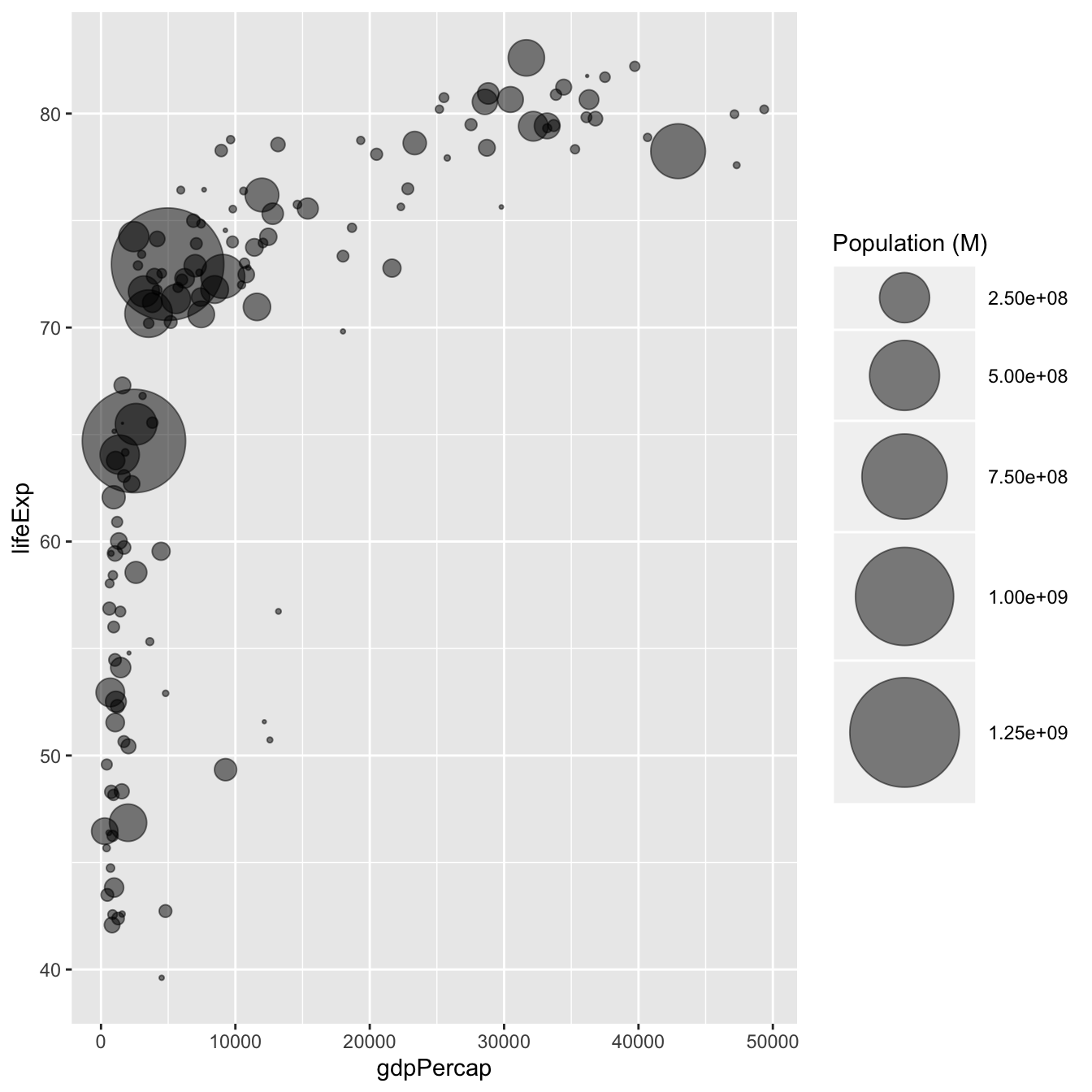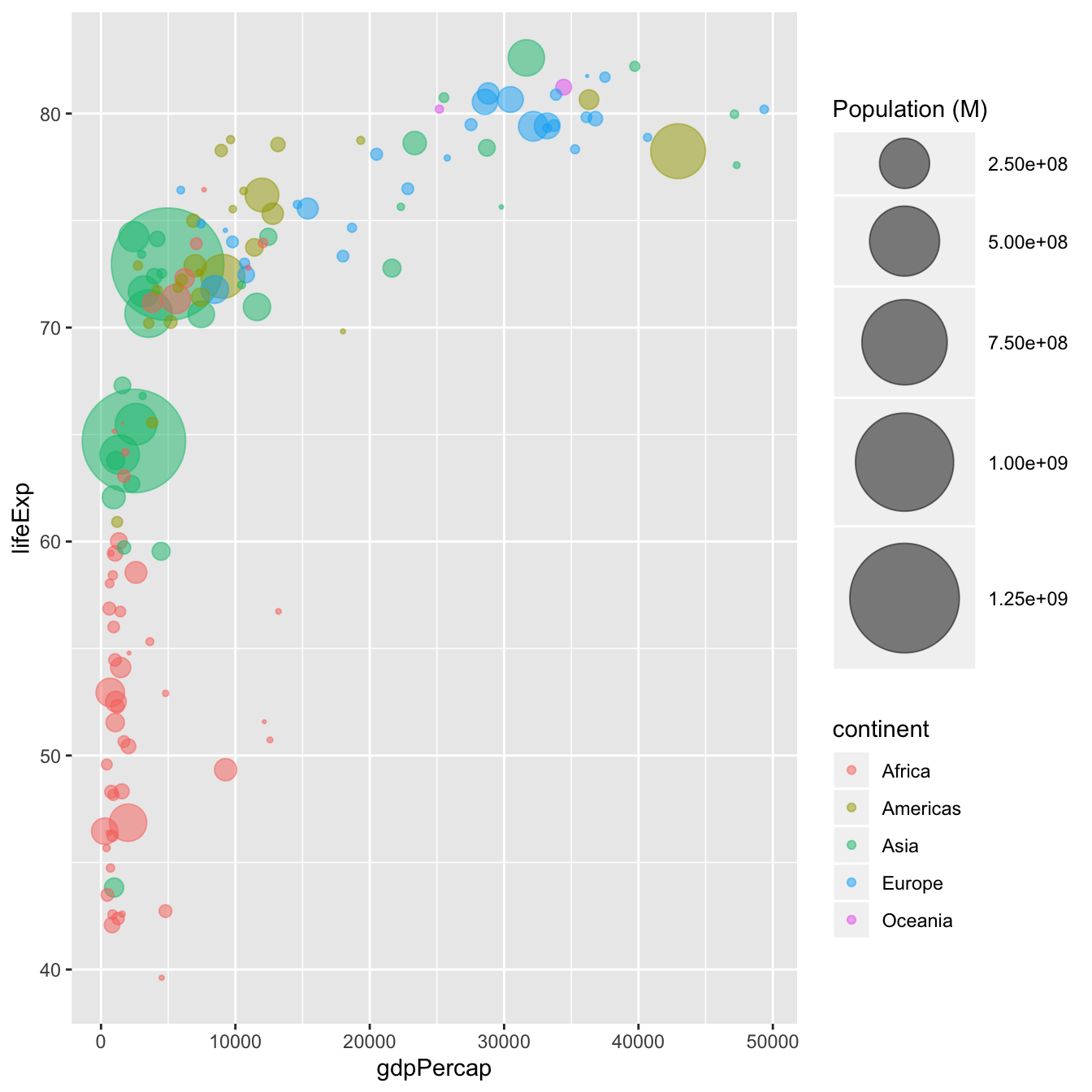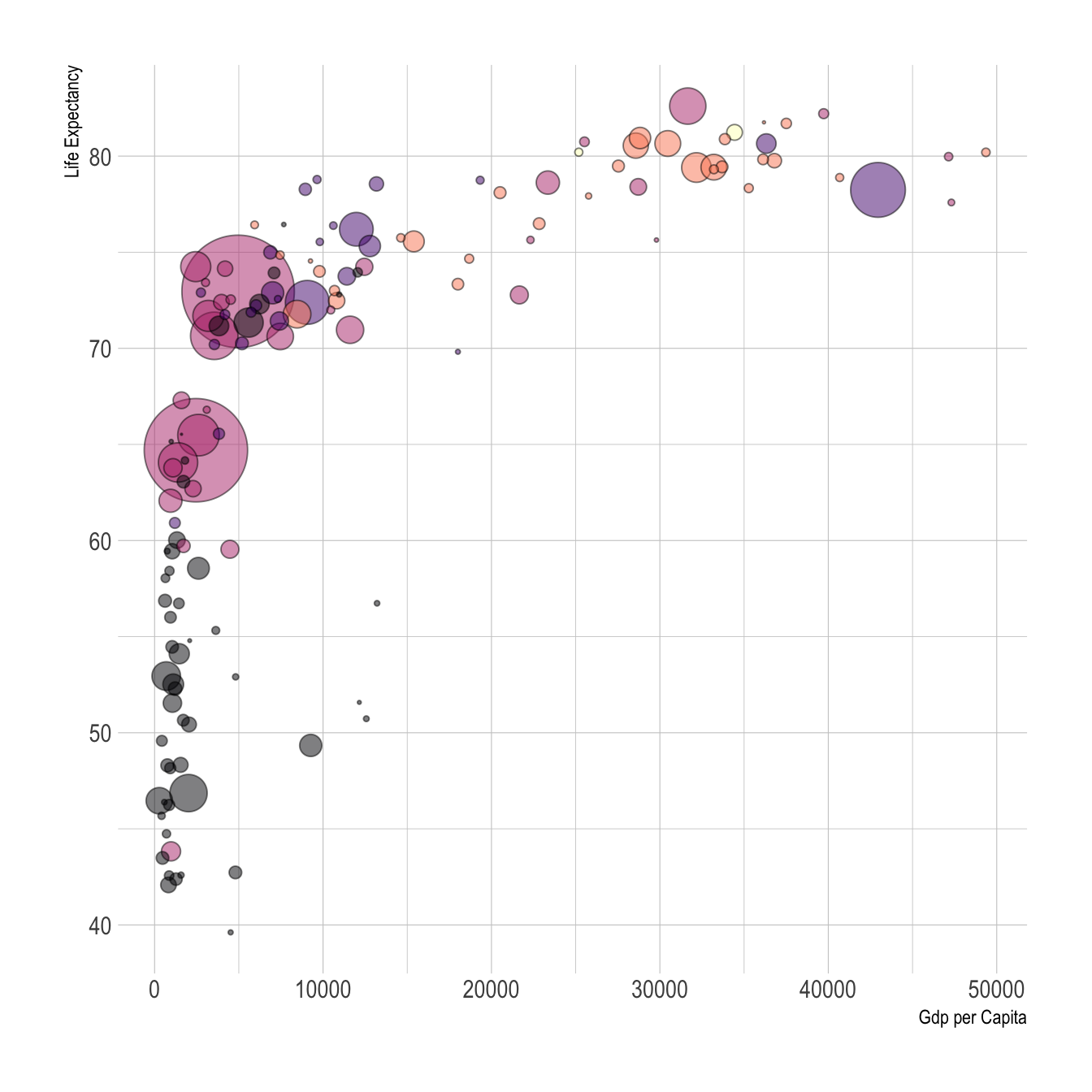# Bubble plot with ggplot2

This post explains how to build a bubble chart with R and ggplot2. It provides several reproducible examples with explanation and R code.

# Most basic bubble chart with `geom_point()`

A bubble plot is a scatterplot where a third dimension is added: the value of an additional numeric variable is represented through the size of the dots. (source: data-to-viz).

With ggplot2, bubble chart are built thanks to the `geom_point()` function. At least three variable must be provided to `aes()`: x, y and size. The legend will automatically be built by ggplot2.

Here, the relationship between life expectancy (`y`) and gdp per capita (`x`) of world countries is represented. The population of each country is represented through circle size.``````# Libraries
library(ggplot2)
library(dplyr)

# The dataset is provided in the gapminder library
library(gapminder)
data <- gapminder %>% filter(year=="2007") %>% dplyr::select(-year)

# Most basic bubble plot
ggplot(data, aes(x=gdpPercap, y=lifeExp, size = pop)) +
geom_point(alpha=0.7)``````

# Control circle size with `scale_size()`

The first thing we need to improve on the previous chart is the bubble size. `scale_size()` allows to set the size of the smallest and the biggest circles using the `range` argument. Note that you can customize the legend name with `name`.

Note: circles often overlap. To avoid having big circles on top of the chart you have to reorder your dataset first, as in the code below.

ToDo: give more details about how to map a numeric variable to circle size. Use of `scale_radius`, `scale_size` and `scale_size_area`. See this post.``````# Libraries
library(ggplot2)
library(dplyr)

# The dataset is provided in the gapminder library
library(gapminder)
data <- gapminder %>% filter(year=="2007") %>% dplyr::select(-year)

# Most basic bubble plot
data %>%
arrange(desc(pop)) %>%
mutate(country = factor(country, country)) %>%
ggplot(aes(x=gdpPercap, y=lifeExp, size = pop)) +
geom_point(alpha=0.5) +
scale_size(range = c(.1, 24), name="Population (M)")``````

# Add a fourth dimension: color

If you have one more variable in your dataset, why not showing it using circle color? Here, the continent of each country is used to control circle color:``````# Libraries
library(ggplot2)
library(dplyr)

# The dataset is provided in the gapminder library
library(gapminder)
data <- gapminder %>% filter(year=="2007") %>% dplyr::select(-year)

# Most basic bubble plot
data %>%
arrange(desc(pop)) %>%
mutate(country = factor(country, country)) %>%
ggplot(aes(x=gdpPercap, y=lifeExp, size=pop, color=continent)) +
geom_point(alpha=0.5) +
scale_size(range = c(.1, 24), name="Population (M)")``````

# Make it pretty

A few classic improvement:

• use of the `viridis` package for nice color palette
• use of `theme_ipsum()` of the `hrbrthemes` package
• custom axis titles with `xlab` and `ylab`
• add stroke to circle: change `shape` to 21 and specify `color` (stroke) and `fill```````# Libraries
library(ggplot2)
library(dplyr)
library(hrbrthemes)
library(viridis)

# The dataset is provided in the gapminder library
library(gapminder)
data <- gapminder %>% filter(year=="2007") %>% dplyr::select(-year)

# Most basic bubble plot
data %>%
arrange(desc(pop)) %>%
mutate(country = factor(country, country)) %>%
ggplot(aes(x=gdpPercap, y=lifeExp, size=pop, fill=continent)) +
geom_point(alpha=0.5, shape=21, color="black") +
scale_size(range = c(.1, 24), name="Population (M)") +
scale_fill_viridis(discrete=TRUE, guide=FALSE, option="A") +
theme_ipsum() +
theme(legend.position="bottom") +
ylab("Life Expectancy") +
xlab("Gdp per Capita") +
theme(legend.position = "none")``````

# What’s next?

This chart could be much better with annotation, or with an interactive version. Don’t you think it is frustrating not to be able to access country name?

Visit the bubble chart section to see it.

Related chart types

## Contact

This document is a work by Yan Holtz. Any feedback is highly encouraged. You can fill an issue on Github, drop me a message on Twitter, or send an email pasting yan.holtz.data with gmail.com.